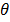# Two forces P and Q of magnitude 2F and 3F, respectively, are at an anglewith each other. If the force Q is doubled, then their resultant also gets doubled. Then, the angle is: (a) 300 (b) 600 (c) 900 (d) 1200

## Question ID - 50249 :- Two forces P and Q of magnitude 2F and 3F, respectively, are at an anglewith each other. If the force Q is doubled, then their resultant also gets doubled. Then, the angle is: (a) 300 (b) 600 (c) 900 (d) 1200

3537

4F2+9F2+12F2cos=R2

4F2+36 F2+24 F2 cos=4R2

4F2+36 F2+24 F2cos=4(13F2+12F2cos)= 52 F2 +48F2coscos==Next Question :
 The actual value resistance R, shown in the figure is 30. This is measured in an experiment as showing using the standard formula R=, where V and I are the readings of the voltmeter and ammeter, respectively. If the measured value of R is 5% less, then the internal resistance of the voltmeter is:(a) 350(b) 570(c) 35(d) 600